# ISEE Middle Level Math Practice Test 14

### Test Information11 questions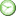0 minutes

Take more free ISEE Mathematics Achievement Practice Tests available from crackssat.com.

1. 12 ÷ 3¼ =

• A.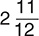• B.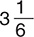• C.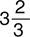• D.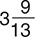2. If 8y + 5 = 41, y =

• A. 4.2
• B. 4.5
• C. 5.1
• D. 5.5

3. (8 + 2)2 – 23 × (5 – 3) =

• A. 84
• B. 86
• C. 92
• D. 104

4. What is the value of the expression 85.1 – a for a = 26.3?

• A. 63.1
• B. 61.4
• C. 60.2
• D. 58.8

5. The melting point of oxygen is −218°C. Its boiling point is only 35°C higher. What is the boiling point of oxygen?

• A. −253°C
• B. −183°C
• C. 183°C
• D. 253°C

6. 30 =

• A. −3
• B. −1
• C. 1
• D. 3

7. Marlene bought a hat and a scarf. The hat cost \$5.96 more than the scarf. The total for the two items, before tax, was \$63.94. What was the cost of the hat?

• A. \$26.39
• B. \$26.99
• C. \$28.99
• D. \$34.95

8. Order from least to greatest: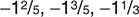• A.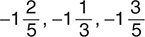• B.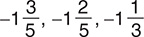• C.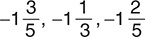• D.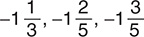9.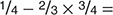• A.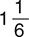• B.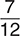• C.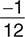• D.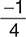10. Hideki drove the 81 miles from Glendale to Roseville in 1½ hours. What was his average speed?

• A. 54 mph
• B. 56 mph
• C. 57 mph
• D. 58 mph

11. The half-life of a radioactive element is 1 second. If you start with 32 grams of it, how much will be left in 4 seconds?

• A. 2 g
• B. 4 g
• C. 8 g
• D. 16 g